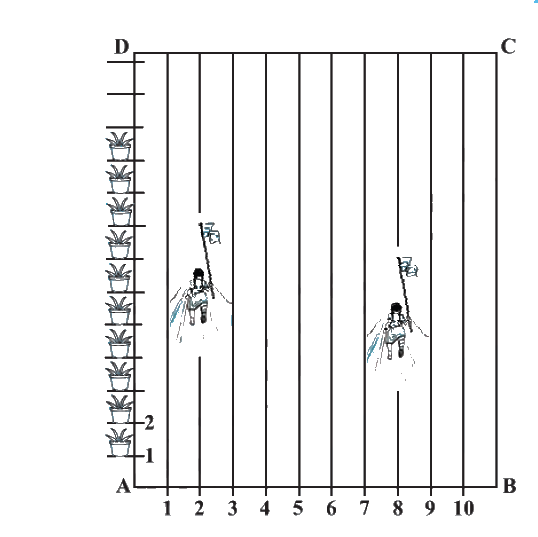In the verge of coronavirus pandemic, we are providing FREE access to our entire Online Curriculum to ensure Learning Doesn't STOP!

# Ex.7.2 Q3 Coordinate Geometry Solution - NCERT Maths Class 10

Go back to  'Ex.7.2'

## Question

To conduct Sports Day activities, in your rectangular shaped school ground $$ABCD$$, lines have been drawn with chalk powder at a distance of $$1\rm\,m$$ each. $$100$$ flower pots have been placed at a distance of $$1\rm\,m$$ from each other along $$AD$$, as shown in the following figure. Niharika runs \begin{align}\frac{1}{4}\end{align}th the distance $$AD$$ on the $$2^\rm {nd}$$ line and posts a green flag. Preet runs \begin{align}\frac{1}{5}\end{align}th the distance $$AD$$ on the eighth line and posts a red flag. What is the distance between both the flags? If Rashmi has to post a blue flag exactly halfway between the line segments joining the two flags, where should she post her flag?Video Solution
Coordinate Geometry
Ex 7.2 | Question 3

## Text Solution

Reasoning:

The coordinates of the point $$P(x, y)$$ which divides the line segment joining the points $$A(x_1, y_1)$$ and $$B(x_2, y_2)$$, internally, in the ratio $$m_1 : m_2$$ is given by the Section Formula.

What is Known?

• The school ground $$ABCD$$ is rectangular shaped.
• Lines are drawn at a distance of $$1\rm\,m$$ each and $$100$$ flower pots have been placed at a distance of $$1\rm\,m$$ each along $$AD$$.
• The distance covered by Niharika and Preet on line $$AD$$.

What is Unknown?

• The distance between the flags posted by Niharika and Preet.
• The position on the line segment where Rashmi has to post the flag.

Steps:

From the Figure,

Given,

• By observation, that Niharika posted the green flag at of the distance $$P$$ i.e., \begin{align}\left( {\frac{1}{4} \times 100} \right)m = 25\,m\end{align} from the starting point of $$2^\rm{nd}$$ Therefore, the coordinates of this point $$P$$ is $$(2, 25)$$.
• Similarly, Preet posted red flag at $$\frac{{1}}{5}$$ of the distance $$Q$$ i.e., \begin{align}\left( {\frac{1}{5} \times 100} \right)m = 20\,m\end{align} from the starting point of $$8^\rm {th}$$ Therefore, the coordinates of this point $$Q$$ are $$(8, 20)$$

We know that the distance between the two points is given by the Distance Formula,

\begin{align}&\sqrt {{{\left( x_1 - x_2 \right)^2 + \left(y_1 - y_2 \right)}^2}} \;\;\dots(1)\end{align}

To find the distance between these flags $$PQ$$ by substituting the values in Equation (1),

\begin{align}PQ &= \sqrt {{{(8 - 2)}^2} + {{(25 - 20)}^2}} \\ &= \sqrt {36 + 25} \\ &= \sqrt {61}\text{ m} \end{align}

• The point at which Rashmi should post her blue flag is the mid-point of the line joining these points.
• Let this point be $$M \;(x, y)$$.

By Section formula

\begin{align} P(x,y)& \!=\! \left[ {\frac{{mx_2 \! + \! nx_1}}{{m \! + \! n}}\!,\!\frac{{my_2 \! + \! ny_1}}{{m \! + \! n}}} \!\right]\;\;\dots(2) \; \end{align}

\begin{align}x &= \frac{{2 + 8}}{2}, \qquad y = \frac{{25 + 20}}{2}\\x &= \frac{{10}}{2}, \qquad\quad\; y = \frac{{45}}{2}\\x &= 5, \qquad\qquad \;y = 22.5\end{align}

Therefore, Rashmi should post her blue flag at $$22.5\;\rm m$$ on $$5^\rm {th}$$ line

Learn from the best math teachers and top your exams

• Live one on one classroom and doubt clearing
• Practice worksheets in and after class for conceptual clarity
• Personalized curriculum to keep up with school